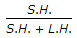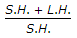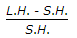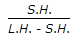# Mechanical Engineering - Heat Transfer, Refrigeration and Air Conditioning - Discussion

1.

Sensible heat factor is given by (where S.H. = Sensible heat, and L.H. = Latent heat)

 [A].[B].[C].[D].Explanation:

No answer description available for this question.

 Ganeshr said: (Feb 15, 2021) It is the ratio of sensible heat to total heat. Total heat = latent heat + sensible heat.